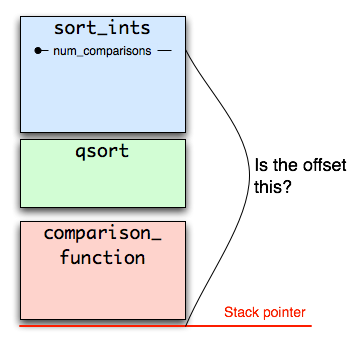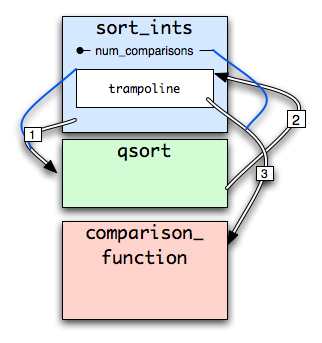Nest
February 5th, 2006
 aaron: Howdy George.
 george: Hey Aaron! How's the C programming coming?
 aaron: Not so good. It doesn't support all the things I'm used to, from other programming languages. Where's the garbage collection? Where's the type safety? Where's the lexically scoped functions?
 george: Yeah, ISO standard C is pretty anemic. Wait, lexus-what?
 aaron: Lexically scoped functions. You know, nested functions - the ability to put a function inside another function. Lots of fancy functional languages can do that, and I wish it were possible in C.
 george: Oh, right. Well, did you know that GCC supports nested functions as an extension? You can call a nested function inside its parent function, and you can also pass a pointer to the nested function to qsort() and other functions. And the nested function can access the local variables of its parent function. Like, say we wanted to sort a bunch of ints with qsort(), but no other function will need to use the comparison function, AND we want to keep track of how many times the comparison function gets called. That's really easy with a nested function: ```int sort_ints(int* array, int count) { int num_comparisons = 0; int comparison_function(const void* a, const void* b) { int first = *(const int*)a; int second = *(const int*)b; num_comparisons++; /* look, we're accessing a variable from our parent function */ if (first < second) return -1; else return first > second; } qsort(array, count, sizeof *array, comparison_function); return num_comparisons; } ``` See? comparison_function() is defined inside sort_ints(). Pretty easy.
 aaron: Hang on. When I try to compile it, this is what happens: ```prompt ) cc -c nested_function.c nested_function.c: In function 'sort_ints': nested_function.c:5: error: nested functions are not supported on MacOSX ```
george: Wha? Nested functions not supported? That's never happened before. In fact, I think that's new with the latest developer tools release from Apple. Does it still work with gcc 3.3? Yes, it seems to. I wonder what changed? Why would Apple stop supporting this feature? Maybe taking a look at how nested functions (used to) work will give us some clues.

So the tricky part of nested functions is accessing the variables in the containing function, such as num_comparisons in the above example. This is because the nested function could be called by some other function (through a pointer) at any point up the stack, so the variable could be at any offset from the stack pointer.How does comparison_function() determine this offset so it knows where to find num_comparisons? Well, it can't, because any number of bizarre functions could be called between sort_ints() and comparison_function(). The stack frame of sort_ints() has to be passed to comparison_function(), as a parameter, so that it can access num_comparisons.

Uh-oh, that sounds like trouble. qsort() is as dumb as a box of granola. It doesn't know anything about extra parameters that have to be passed to comparison_function(). All it has is the function pointer, which is just an address in memory. How will comparison_function() get the stack frame containing num_comparisons, then?

Maybe it could be put in a global variable? But no, that won't work - sort_ints() could be called recursively, so there could be multiple sort_ints() stack frames that we need to keep track of at once.

What if, instead of handing qsort() a pointer to the comparison_function() code, we instead passed a pointer to some data structure containing the stack frame of sort_ints() AND the address of comparison_function()? That data structure could turn around and call comparison_function() passing it the stack frame it needs. And the data structure could live on the sort_ints() stack, so we would have one per invocation of sort_ints(), which is just what we need.

But again, qsort() is as dumb as a baked potato. It wants to jump to whatever address we give it. So our "data structure" will have to be real, executable code that does this dirty work. Yes, we will have to generate executable code at runtime. Who wants to play compiler?

Hmm, this is as clear as cola. Maybe a diagram will help.1. sort_ints() passes the address of the generated code ("trampoline") to qsort.
2. qsort() jumps to the address of the generated code, thinking it's the real function! Ha ha ha!
3. The generated code calculates the address of num_comparisons by subtracting a little from the instruction pointer, and then calls comparison_function(), passing it that address.
Believe it or not, this is what gcc actually does (did). Let's take a simpler example so we can look at the code that gcc generates. Because gcc-3.3, which still supports nested functions, is only available on the PowerPC in OS X, we'll look at PowerPC code.

Here's some simple nested-function code:

```void extern_function(void (*func_ptr)(void));
void outer_function(void) {
int x = 0;
void inner_function(void) {
x++;
}
extern_function(inner_function);
}
```
Here's how we compile it. -static cuts through the position-independent code haze, making it clearer what's going on:
```prompt ) gcc-3.3 -Os -static -S nested_function.c
```
Here's what we get:
```_inner_function.0:
stw r11,-16(r1)
lwz r2,0(r11)
stw r2,0(r11)
blr
_outer_function:
mflr r0
lis r5,ha16(_inner_function.0)
stw r0,8(r1)
la r5,lo16(_inner_function.0)(r5)
stwu r1,-128(r1)
li r4,40
bl ___trampoline_setup
li r0,0
stw r0,64(r1)
bl _extern_function
lwz r0,136(r1)
 george: That's right. Note that while the C code for inner_function() is inside outer_function(), the assembly functions aren't nested. See that ___trampoline_setup business in outer_function()? That's where the runtime code generation occurs. Here's where ___trampoline_setup is defined. Let's see what it does. ```/* R3 = stack address to store trampoline */ /* R4 = length of trampoline area */ /* R5 = function address */ /* R6 = static chain */ .globl ___trampoline_setup ___trampoline_setup: mflr r0 /* save return address */ bcl 20,31,LCF0 /* load up __trampoline_initial into r7 */ LCF0: mflr r11 addis r7,r11,ha16(LTRAMP-LCF0) lg r7,lo16(LTRAMP-LCF0)(r7) subi r7,r7,4 li r8,trampoline_size /* verify trampoline big enough */ cmpg cr1,r8,r4 srwi r4,r4,2 /* # words to move (insns always 4-byte) */ addi r9,r3,-4 /* adjust pointer for lgu */ mtctr r4 blt cr1,Labort mtlr r0 ``` That code does some setup and also checks that the caller allocated enough space for the generated code (the "trampoline"). If something weird were to happen, such as the compiler getting out of sync with libc, then the dynamically generated code could produce a buffer overflow - this makes sure that doesn't happen. ``` /* Copy the instructions to the stack */ Lmove: lwzu r10,4(r7) stwu r10,4(r9) bdnz Lmove ``` Most of the generated code is boilerplate (thankfully). In fact, the only non-boilerplate code is the address of the nested function and something called the "static chain," which I don't understand (yet). In that code, we are just copying the boilerplate from where its "prototype" lives into our block of instructions on the stack. ``` /* Store correct function and static chain */ stg r5,Lfunc(r3) stg r6,Lchain(r3) ``` Our dynamic code generation is all of two instructions. In that code, we stick the address of the nested function into the (recently copied) code block. ``` /* Now flush both caches */ mtctr r4 Lcache: icbi 0,r3 dcbf 0,r3 addi r3,r3,4 bdnz Lcache /* Finally synchronize things & return */ sync isync blr ``` The PowerPC is picky about making you flush the instruction cache after changing memory that contains instructions. This is normally no problem, since it's a very infrequent operation; in fact, it may even provide some protection against buffer overflow exploits (since you might have to flush the instruction cache before it will see the evil instructions you wrote). But here we have a legitimate reason to write instructions, so we flush the cache. As an interesting side note, we jump through this hoop to get at the stack frame for the outer function. But we never actually have to store the stack frame in our dynamically generated code because the code itself lives on the stack. That is, the code just asks itself for the instruction pointer, and because the code is in the stack frame of the outer function, the instruction pointer points to exactly the place we want (after a bit of arithmetic). The location of the generated code is as important as the code itself.